## Example Questions

### Example Question #1 : How To Find Compound Interest

A five-year bond is opened with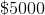in it and an interest rate of%, compounded annually. This account is allowed to compound for five years. Which of the following most closely approximates the total amount in the account after that period of time?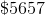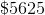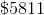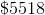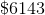Explanation:

Each year, you can calculate your interest by multiplying the principle () by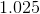. For one year, this would be: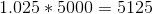For two years, it would be:, which is the same as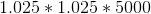Therefore, you can solve for a five year period by doing: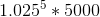Using your calculator, you can expand the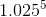into a series of multiplications. This gives you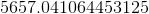, which is closest to### Example Question #13 : Gre Quantitative Reasoning

Jack has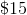,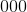to invest. If he invests two-thirds of it into a high-yield savings account with an annual interest rate of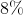, compounded quarterly, and the other third in a regular savings account at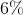simple interest, how much does Jack earn after one year?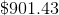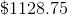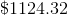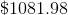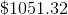Explanation:

First, break the problem into two segments: the amount Jack invests in the high-yield savings, and the amount Jack invests in the simple interest account (10,000 and 5,000 respectively).

Now let's work with the high-yield savings account. $10,000 is invested at an annual rate of 8%, compounded quarterly. We can use the compound interest formula to solve: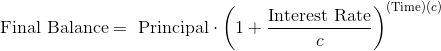Plug in the values given: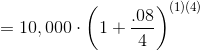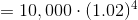Therefore, Jack makes$824.32 off his high-yield savings account. Now let's calculate the other interest: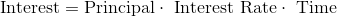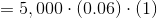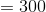Add the two together, and we see that Jack makes a total of,off of his investments.

### Example Question #2 : How To Find Compound Interest

If a cash deposit account is opened with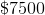for a three year period at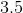% interest compounded once annually, which of the following is closest to the positive difference between the interest accrued in the third year and the interest accrued in the second year?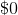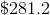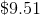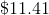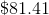Explanation:

It is easiest to break this down into steps. For each year, you will multiply by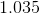to calculate the new value. Therefore, let's make a chart:

After year 1: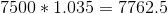; Total interest: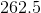After year 2: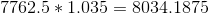; Let us round this to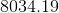; Total interest: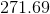After year 3: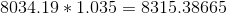; Let us round this to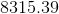; Total interest: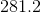Thus, the positive difference of the interest from the last period and the interest from the first period is: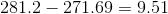Tired of practice problems?

Try live online GRE prep today.